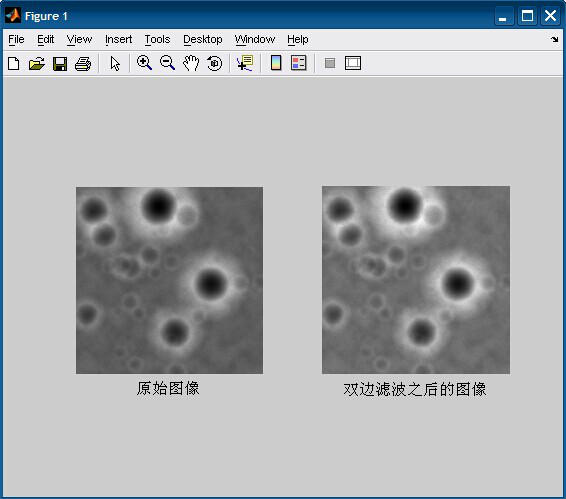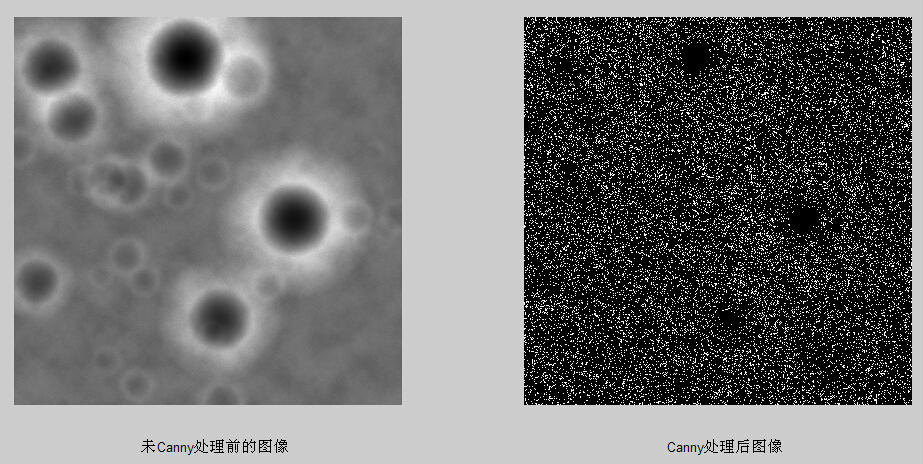• 三天的比赛结束了。今年的题比较变态，我已经被虐到无力吐槽了。。...没想到接触到图像处理是在数学建模的比赛。。。第二天和第三天啥也没干，就在死抠图像处理程序。最终在第三天晚上完美解决。
 三天的比赛结束了。今年的题比较变态，我已经被虐到无力吐槽了。。不过真是不到最后逼自己一把成不了事。最后一天突破了好多的困难。在最后的一小时里写完了论文，完成了全部的任务。    一开始报选修课的时候选matlab的原因是想学一下matlab的图像处理。然后课上一直讲的是基础。。没想到接触到图像处理是在数学建模的比赛中。。。第二天和第三天啥也没干，就在死抠图像处理程序。最终在第三天晚上完美解决。从第一天搜集的大量文献中总结筛选，最终决定了“原始照片 → 双边滤波处理（去噪）→ 切割分块取均值后重组图像 → Canny算子边缘检测 → 对比”的最佳线路。
双边滤波：程序：

1
2
3
4
5
6
7
8
9
10
11
12
13
14
15
16
17
18
19
20
21
22
23
24
25
26
27
28
29
30
31
32
33
34
35
36
37
38
39
40
41
42
43
44
45
46

%%%%%%%%图5.6程序%%%%%%%%%
clear all;
close all;
clc;
img=imread('附件3 距2400m处的数字高程图.tif');
% img=mat2gray(img);%2值化
 
%%%%%%%%%%第一个图：显示原始图像%%%%%%%%%%%%
subplot(121);
imshow(img);
title('原始图像','position',[1150,2600]);
%%%%%%%%%%%%%%%%%%%%%%%%%%%%%%%%%%
 
%%%%%%%%%%%%%%%%%%%%%双边滤波%%%%%%%%%%%%%%%%%%%%%%%
r=10;        %模板半径
[m n]=size(img);
imgn=zeros(m+2*r+1,n+2*r+1);
imgn(r+1:m+r,r+1:n+r)=img;
imgn(1:r,r+1:n+r)=img(1:r,1:n);                 %扩展上边界
imgn(1:m+r,n+r+1:n+2*r+1)=imgn(1:m+r,n:n+r);    %扩展右边界
imgn(m+r+1:m+2*r+1,r+1:n+2*r+1)=imgn(m:m+r,r+1:n+2*r+1);    %扩展下边界
imgn(1:m+2*r+1,1:r)=imgn(1:m+2*r+1,r+1:2*r);       %扩展左边界
sigma_d=2;
sigma_r=0.1;
[x,y] = meshgrid(-r:r,-r:r);
w1=exp(-(x.^2+y.^2)/(2*sigma_d^2));     %以距离作为自变量高斯滤波器
h=waitbar(0,'图像双边滤波中...');
for i=r+1:m+r
    for j=r+1:n+r

        w2=exp(-(imgn(i-r:i+r,j-r:j+r)-imgn(i,j)).^2/(2*sigma_r^2)); %以周围和当前像素灰度差值作为自变量的高斯滤波器
        w=w1.*w2;
        s=imgn(i-r:i+r,j-r:j+r).*w;
        imgn(i,j)=sum(sum(s))/sum(sum(w));
    end
    waitbar(i/m);
end
close(h)
%%%%%%%%%%%%%%%%%%%%%%%%%%%%%%%%%%%%%%%%%%%
 
%%%%%%%%%%%%%%第二个图：滤波后图像%%%%%%%%%%%%%%%%%
% figure;
img1=mat2gray(imgn(r+1:m+r,r+1:n+r));
subplot(122);
imshow(img1);
title('双边滤波之后的图像','position',[1160,2600]);
%%%%%%%%%%%%%%%%

Canny算子边缘检测程序：

1
2
3
4
5
6
7
8
9
10
11
12
13
14
15
16
17
18
19
20
21
22
23
24
25
26
27
28
29
30
31
32
33
34
35
36
37
38
39
40
41
42
43
44
45
46
47
48
49
50
51
52

%%%%%%%%%%%%%%%%%图5.7程序%%%%%%%%%%%%%%%%%%%%%%%%%%%
clear all;
close all;
clc;
img=imread('附件3 距2400m处的数字高程图.tif');
% img=mat2gray(img);%2值化
 
%%%%%%%%%%%%%%%%%%%%%双边滤波%%%%%%%%%%%%%%%%%%%%%%%
r=10;        %模板半径
[m n]=size(img);
imgn=zeros(m+2*r+1,n+2*r+1);
imgn(r+1:m+r,r+1:n+r)=img;
imgn(1:r,r+1:n+r)=img(1:r,1:n);                 %扩展上边界
imgn(1:m+r,n+r+1:n+2*r+1)=imgn(1:m+r,n:n+r);    %扩展右边界
imgn(m+r+1:m+2*r+1,r+1:n+2*r+1)=imgn(m:m+r,r+1:n+2*r+1);    %扩展下边界
imgn(1:m+2*r+1,1:r)=imgn(1:m+2*r+1,r+1:2*r);       %扩展左边界
sigma_d=2;
sigma_r=0.1;
[x,y] = meshgrid(-r:r,-r:r);
w1=exp(-(x.^2+y.^2)/(2*sigma_d^2));     %以距离作为自变量高斯滤波器
h=waitbar(0,'图像双边滤波中...');
for i=r+1:m+r
    for j=r+1:n+r

        w2=exp(-(imgn(i-r:i+r,j-r:j+r)-imgn(i,j)).^2/(2*sigma_r^2)); %以周围和当前像素灰度差值作为自变量的高斯滤波器
        w=w1.*w2;
        s=imgn(i-r:i+r,j-r:j+r).*w;
        imgn(i,j)=sum(sum(s))/sum(sum(w));
    end
    waitbar(i/m);
end
close(h)
%%%%%%%%%%%%%%%%%%%%%%%%%%%%%%%%%%%%%%%%%%%
 
%%%%%%%%%%%%%%第二个图：滤波后图像%%%%%%%%%%%%%%%%%
% figure;
img1=mat2gray(imgn(r+1:m+r,r+1:n+r));
subplot(121);
imshow(img1);
title('未Canny处理前的图像','position',[1200,2600])
%%%%%%%%%%%%%%%%%%%%%%%%%%%%%%%%%%%%%%%%%%%%%%%%
 
 
%%第四个图：第二个图滤波后图像的canny边缘检测处理结果%%
% canny边缘检测
h2=waitbar(0,'绘制双边滤波之后的canny图...');
img4 = edge(img1,'canny');  % 调用canny函数
waitbar(3);
subplot(122)
imshow(img4);  % 显示分割后的图像，即梯度图像
title('Canny处理后图像','position',[1200,2600])
close(h2);
%%%%%%%%%%%%%%%%%%%%%%%%%%%%%%%%%%%%%%%%%%%%%%%%%%%

由上图可以看出，处理效果并不好。我们进行切割分块取均值后再还原图像的方法处理后再进行Canny算子边缘检测。
图片：程序：

1
2
3
4
5
6
7
8
9
10
11
12
13
14
15
16
17
18
19
20
21
22
23
24
25
26
27
28
29
30
31
32
33
34
35
36
37
38
39
40
41
42
43
44
45
46
47
48
49
50
51
52
53
54
55
56
57
58
59
60
61
62
63
64
65
66
67
68
69
70
71
72
73
74

%%%%%%%%%%%%%%%%图5.8程序%%%%%%%%%%%%%%%%%%%%%%%%%%%%
clear all;
close all;
clc;
img=imread('附件3 距2400m处的数字高程图.tif');
 
 
%%%%%%%%%%%%%%%%%%%%%双边滤波%%%%%%%%%%%%%%%%%%%%%%%
r=10;        %模板半径
[m n]=size(img);
imgn=zeros(m+2*r+1,n+2*r+1);
imgn(r+1:m+r,r+1:n+r)=img;
imgn(1:r,r+1:n+r)=img(1:r,1:n);                 %扩展上边界
imgn(1:m+r,n+r+1:n+2*r+1)=imgn(1:m+r,n:n+r);    %扩展右边界
imgn(m+r+1:m+2*r+1,r+1:n+2*r+1)=imgn(m:m+r,r+1:n+2*r+1);    %扩展下边界
imgn(1:m+2*r+1,1:r)=imgn(1:m+2*r+1,r+1:2*r);       %扩展左边界
sigma_d=2;
sigma_r=0.1;
[x,y] = meshgrid(-r:r,-r:r);
w1=exp(-(x.^2+y.^2)/(2*sigma_d^2));     %以距离作为自变量高斯滤波器
h=waitbar(0,'图像双边滤波中...');
for i=r+1:m+r
    for j=r+1:n+r

        w2=exp(-(imgn(i-r:i+r,j-r:j+r)-imgn(i,j)).^2/(2*sigma_r^2)); %以周围和当前像素灰度差值作为自变量的高斯滤波器
        w=w1.*w2;
        s=imgn(i-r:i+r,j-r:j+r).*w;
        imgn(i,j)=sum(sum(s))/sum(sum(w));
    end
    waitbar(i/m);
end
close(h)
 
%%%%%%%%%%%%%%第二个图：滤波后图像%%%%%%%%%%%%%%%%%
img1=mat2gray(imgn(r+1:m+r,r+1:n+r));
 
 
%%%%%%%%%%滤波后图像高程值分块单位化并求均值%%%%%%%%%
a=zeros(575);
b=zeros(575);
c=zeros(575);
d=zeros(575);
H=waitbar(0,'滤波后图像高程值分块单位化并求均值...');%粗壁障，采用4*4计算单位。
for i=1:1:575;
    for j=1:1:575;
        a(j,i)=img1(4*j-1,4*i-1);
        b(j,i)=img1(4*j-1,4*i);
        c(j,i)=img1(4*j,4*i-1);
        d(j,i)=img1(4*j,4*i);
        a1=a(j,i);b1=b(j,i);c1=c(j,i);d1=d(j,i);
        img2(j,i)=(a1+b1+c1+d1)/16;% 取平均数之后
    end
    waitbar(i/575);
end
close(H);
%%%%%%%%%%%%%%%%%%%%%%%%%%%%%%%%%%%%%%%%%%%%%%%%%
 
 
%%%%第三个图：滤波后图像高程值分块单位化后均值排列图%%%%%%
% figure(1);
% imagesc(img1);
% figure(2);
subplot(121)
imshow(img2);
title('未Canny处理前的图像','position',[287,630])
 
 
 
%%%%%%第五个图：第三个图的canny边缘检测处理结果%%%%%%%
% canny边缘检测
img5 = edge(img2,'canny');  % 调用canny函数
subplot(122);
imshow(img5);  % 显示分割后的图像，即梯度图像
title('Canny处理后图像','position',[287,635])
%%%%%%%%%%%%%%%%%%%%%%%%%%%%%%%%%%%%%%%%%%%%%%%%%%%


展开全文• 如何入门参与数学建模 2019-05-21 13:23:26
数学建模感想 纪念逝去的大学数学建模：两次校赛，两次国赛，两次美赛，一次电工杯。从大一下学期组队到现在，大三下学期，时间飞逝，我的大学建模生涯也告一段落。感谢建模路上帮助过我的学长和学姐们，滴水之恩当...大学生数学建模
• 建议按照课程顺序学习，帮助大家更好的掌握该领域的应用和知识，并在实战编程深入学习和开展研究。相信经过努力学习和反复实践，可以取得收获。matlab matlab安装教程 matlab图像处理 bp神经网络matlab实例
• 数学建模问题分析 2020-04-13 23:00:34
数据处理 1）插值拟合：对数据补全和基本趋势分析 对数据补全和基本趋势分析（根据某个未知函数（或已知但难于求解的函数）的几个已知数据点求出变化规律和特征相似的近似曲线的过程。） 插值：求过已知有限个数据点...数学建模
• 数学建模算法与应用》方法概述序言：学习司守奎《数学建模算法与应用》第2版过程，给出一个内容的提纲，希望可以在回忆复习时快速建立知识结构。包含了数学建模所需用到的一些方法和常见问题类型。 其中前半...数学建模 算法 matlab
• 数学建模中四大模型总结 2019-06-13 11:40:03
1.1数学规划模型 线性规划、整数线性规划、非线性规划、多目标规划、动态规划。 1.2微分方程组模型 阻滞增长模型、SARS传播模型。 1.3图论与网络优化问题 最短路径问题、网络最大流问题、最小费用最大流问题、...数学建模
• 通常来说，图像的退化可以概括为初始的输入图像f(x,y)f(x,y)，经过退化函数k(x,y)k(x,y)作用后，再附加上随机噪声n(x,y)n(x,y)，最后输出为模糊图像g(x,y)g(x,y)。 用数学形式描述为：g(x,y)=k(x,y)⨂f(x,y)+n(x,y)...
• 数学建模心得 2019-03-12 09:10:35
这篇文章是本人的数学建模队友在建模过程的一些心得，联系作者请留言，未经允许严禁转载 1、数学建模的定义   数学建模就是根据实际问题来建立数学模型，对数学模型来进行求解，然后根据结果去解决实际问题。当...数学建模
• 数字图像处理之图像复原 2018-02-26 17:58:04
一、图像复原与图像增强的区别图像的增强是一个主观的过程，其目的是改善图片的质量，对感兴趣的部分加以增强，对不感兴趣的部分予以抑制。...课本图像退化过程建模为一个退化函数和一个加性噪声...matlab编程
• 笔者在18年五月底参加了济南大学第七届数学建模校赛，现在将论文贴出，在博文，将整理出论文核心的详细知识点 基于 MATLAB 的数字图像处理模型 1 摘要 本文利用 MATLAB 等工具对题目给出图像进行数字化处理，...图像处理 数学建模
• （1）基于阈值的分割方法：可在各种颜色空间或不同通道完成阈值、自适应阈值、 （2）基于边缘的分割方法：各种边缘检测算子 （3）基于区域的分割方法：分水岭、区域归并与分裂 （4）图割分割：最大流（最小割）...
• 一份失败的数学建模心得 2019-10-26 19:34:48
一直觉得自己应该写点东西，但又觉得自己还是不够资格，毕竟在数学建模上的建树不多甚至很差。但是还是想把自己的一些经验分享给即将参加国赛的学弟学妹吧。我想在此之前你已经看过很多国赛一等二等大佬们的经验分享...
• 首先，必须注意这里所限制的处理条件。 关于图像退化/复原模型 退化的图像是由成像系统的退化加上额外的噪声形成的。 1.只考虑噪声引起的退化 噪声模型，包含于空间不相关和相关两种，除了空间周期噪声，这里所...
• 说说建模的那些事！ 比赛第一天。。。。。。。。。。。。。。。。。。。。。 一个大一的弱鸡，算第一次参加建模比赛吧！（虽然之前有报过美赛，但是没有写出来，浪费100美元，心疼自己1s），这个比赛是建模会长第二...
• 数学建模中，常常会涉及一些预测类问题。预测方法种类繁多，从经典的单耗法、弹性系数法、统计分析法，到现在的灰色预测法、专家系统法和模糊数学法、甚至刚刚兴起的神经元网络法、优选组合法和小波分析法等200余...
• 我的微信公众号名称：AI研究...  数学建模就是根据实际问题来建立数学模型，对数学模型来进行求解，然后根据结果去解决实际问题。   当需要从定量的角度分析和研究一个实际问题时，人们就要在深入调查研究、了解...
• 数学建模集训知识大纲 2019-09-16 16:17:49
文章目录数学建模集训知识大纲评价算法简单加权法逼近于理想解的排序法(TOPSIS算法)层次分析法主成分分析法模糊综合评价法聚类分析法秩和比法人工神经网络熵权法灰色关联度分析预测算法插值拟合回归模型预测灰色预测...数学建模
• Python图像处理 2016-06-24 12:55:26
第 1 章　基本的图像操作和处理 本章讲解操作和处理图像的基础知识，将通过大量示例介绍处理图像所需的 Python 工具包，并介绍用于读取图像、图像转换和缩放、计算...1.1　PIL：Python图像处理类库 PIL（Pyt
• 该书共有30个MATLAB神经网络的案例(含可运行程序），包括BP、RBF、SVM...还包含PSO(粒子群）、灰色神经网络、模糊网络、概率神经网络、遗传算法优化等内容。该书另有31个配套的教学视频帮助读者更深入地了解神经网络。神经网络
...